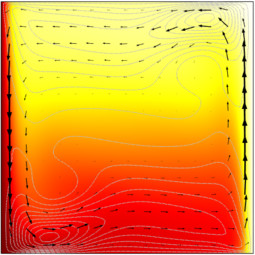# Application Gallery

## Buoyancy Flow of Free Fluids

Application ID: 665

This model couples the Navier Stokes equations and the heat transfer equations to examine density driven flow of free fluids. Here the fluid is in a square cavity with a heated wall. The buoyancy force is a Boussinesq term added to the Navier-Stokes equations. The equation is nondimensionalized, so the material coefficients are set up using Rayleigh and Prandtl numbers.

The parametric solver is used to analyze this problem for increasing Rayleigh number. This is a benchmark problem from G. De Vahl Davis (1983) that has been used to evaluate a number of different hydrodynamic flow codes.This model example illustrates applications of this type that would nominally be built using the following products: